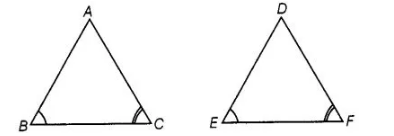# In Δ ABC and ΔDEF, ∠B = ∠E, ∠F = ∠C

Question:

In Δ ABC and ΔDEF, ∠B = ∠E, ∠F = ∠C and AB = 30E Then, the two triangles are

(a) congruent but not similar

(b) similar but not congruent

(c) neither congruent nor similar

(d) congruent as well as similar

Solution:

(b) In ΔABC and ΔDEF, ∠B = ∠E, ∠F = ∠C and AB = 3DEWe know that, if in two triangles corresponding two angles are same, then they are similar by AAA similarity criterion. Also, ΔA8C and ΔDEF do

not satisfy any rule of congruency, (SAS, ASA, SSS), so both are not congruent.